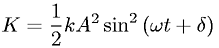Equations > Physics > Oscillations and Waves > Kinetic energy of simple harmonic motion

### Kinetic energy of simple harmonic motionLatex Code:

MathML Code:

 $K=\frac12{\mathrm{kA}}^{2}{\mathrm{sin}}^{2}\left(\omega t+\delta \right)$

MathType 5.0: